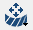# Squeak and Rattle

Allows one to identify squeak and rattle issues at various points along the contact surface of neighboring parts.

Squeak and rattle issues are always caused by relative displacement between two parts which are mounted with or without any gap and might interact for a short duration of time. This means, if there is no relative displacement, then there are no squeak and rattle issues.
Note: Workbench is supported for transient dynamic analysis only.

## Terminology

Rattle
Rattle is caused when there is a relative motion between components that come in contact for a short duration of time, due to impact forces. Rattle generally occurs when the components are loose or overly flexible.
Squeak
Squeak is an undesirable sound that occurs when two surfaces interact under stick-slip phenomenon, and friction induces is caused by a relative motion between the surfaces. The frictional energy is stored in the contact surfaces and is released when it exceeds the kinetic friction, which in turn produces an audible squeak.

## Setup

1. On the main window toolbar, click Squeak and Rattle.
2. In the pop-up dialog, choose the transient subcase to link the Squeak and Rattle Workbench.
3. Enter the failure criteria for Gap tolerance (Open close) and Gap tolerances (Stick slip).
4. Define a set of points at the contact surface of neighboring parts to identity squeak and rattle.
1. In the Squeak and Rattle Workbench tab, click(Add/edit sampling points) icon.
2. Define the sampling points using one of the following approaches.
5. Click the Solveicon to run the analysis.
6. When complete, the analysis provides the following results:
• Final status
• Squeak and rattle: The normal gap is below the open-close tolerance and the tangential distance is above the stick-slip tolerance.
• Rattle: The normal gap is below the open-close tolerance and the tangential distance is below the stick-slip tolerance.
• NA: The normal gap is above the open-close tolerance.
• Initial Gap: Lists the initial gap between the parts at various sampling points before applying the load.
• Minimal gap: Lists the closest normal distance between the parts at various sampling points along the total time span when the parts are loaded.
• Maximum tangential displacement: Lists the maximum displacement tangentially at various sampling points along the total time span when the parts are loaded.

## Examples

A part is considered to have:
• Squeak and rattle if the normal gap is below the open-close tolerance and the tangential distance is above the stick-slip tolerance.
• Rattle if the normal gap is below the open-close tolerance and the tangential distance is below the stick-slip tolerance.
• NA if the normal gap is above the open-close tolerance.

### Example 1

Open-close tolerance = 0

Stick-slip tolerance = 0

Initial gap between the plates = 5mm

Point 1 has both squeak and rattle as the normal gap is below the open-close tolerance and tangential displacement is above the stick-slip tolerance.

Point 2 is green as it has a normal gap much higher than the open-close tolerance. The parts are always separated, so it neither rattles nor squeaks.

### Example 2

Open-close tolerance = 5 mm

Stick-slip tolerance = 0

Initial gap between the plates = 5mm

Point 1 and Point 2 are identified to squeak and rattle as the normal gap is below the open-close tolerance and tangential displacement is above the stick-slip tolerance.

### Example 3

Open-close tolerance = 5

Stick-slip tolerance = 0.1

Initial gap between the plates = 5mm

Point 1 and Point 2 are identified to rattle only as the normal gap is below the open-close tolerance and tangential displacement is below the stick-slip tolerance.

Hence no squeak and the points' final state is set to Rattle only.